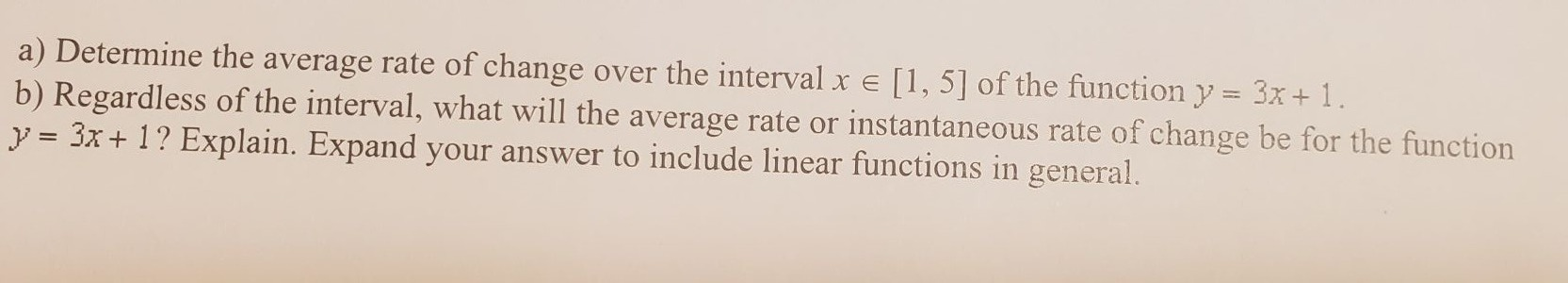### Still have math questions?

Algebra
Questiona) Determine the average rate of change over the interval $$x \in [ 1,5 ]$$ of the function $$y = 3 x + 1$$

b) Regardless of the interval, what will the average rate or instantaneous rate of change be for the function $$y = 3 x + 1$$ ? Explain. Expand your answer to include linear functions in general.